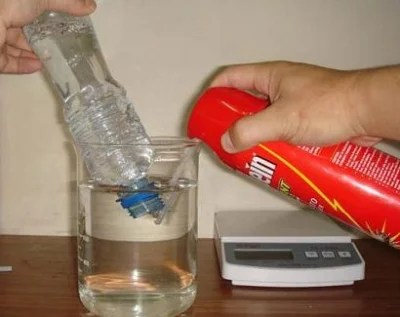How to find out Air’s mass

How to find out Air’s mass
5 (100%) 1 vote[s]

How to find out Air’s mass Level Elementary

Experiment Air’s mass

Concept

The air has mass.Let's measure the air’s mass with a spray can and PET bottle.

Materials

used spray can,  PET bottle (500ml or 1000ml), and air pump, vinyl  nozzle, adhesive tape,  pin for the ball, electronic balance or balance, bond, and water tank

Procedure

1. Confirm that there is no gas in the spray can.
2. The nozzle is attached to the spray can by the bond.
3. The adhesive tape is rolled in the pin for the ball.
4. The pin is inserted to another side of nozzle tightly.
5. The air is put into the spray can enough by the air pump. The spray button is pushed during pumping.
6. Measure the mass of the spray can by the balance.
7. Water is put in the water tank Water is put in the PET bottle, and it is set up inverted in the water tank.
8. The air 1000ml is taken from the spray can by replacing the water of the PET bottle.
9. Measure the mass of the spray can by the balance again.
10. The mass of the air 1000ml is calculated.

Working

The following are one example of the measurement result. The mass of the spray can with air: 117.6g The mass of the can after taking out air1000ml : 116.4g As a result, the mass of air 1000ml (1dm ):1.2g    The mass of air 1dm  in 0℃  1atm is 1.293g.

Question

Let's calculate the mass of air in the classroom.

The mass of the air 1dm3 is assumed to be 1.2g, and the height, width, and length of the classroom are assumed to be 3.5m, 7m, 10m each.

The volume of the classroom=3.5 m ×7m× 10m=245m3 (meter cube)

1m 3=1000 dm3
The mass of air in the classroom=1.2 g×1000×245=294000g =294kgAir’s mass ExperimentAir’s mass experiment